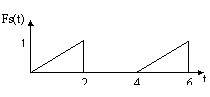# Function of substitution or [ ] operator

## Interest

The function of substitution, represented by the operator [ ], enables to replace in an existing function the value of a parameter by a formula.

This function has several possibilities described in the examples below.

## Definition

The syntax of the (operator [ ]) function of substitution is the following:

PEs = PEi [X = Formula (X, …)]

where:

• PEi is the I/O parameter “carrying” the initial function
• PEs is the I/O parameter “carrying” the function of substitution

The function of substitution PEi[X=Formula(X, ...)] returns the value of the function PEi , where the argument X is replaced by the formula Formula(X, ...).

## Operation mode

The operation mode is presented on an example of a transient application.

We suppose that the following initial function was already created: PEi = Trapez(TIME, T1 ,T2 ,T3)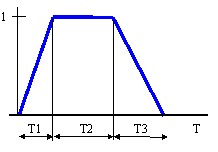Create an I/O parameter: PEs = PEi[TIME=TIME-Td]

where:

• TIME is the time parameter
• Td is the value of time delay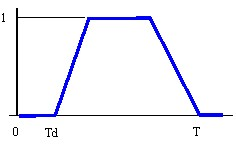## Examples

Examples of using the substitution function are presented below.

Examples

Initial function:

Fi(t) = Trapez(t,2,0,0)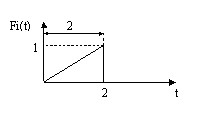The function of substitution:

Fs(t) = Fi(t)[t=t-2]

delay with 2 seconds the initial function Fi(t)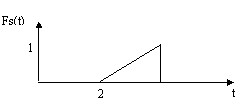The function of substitution:

Fs(t) = Fi(t)[t=t*2]

increases two times the time of initial function Fi(t)The function of substitution:

Fs(t) = Fi(t)[t=Modulo(t,4)]

periodize the initial function Fi(t) with the period of 4 seconds.